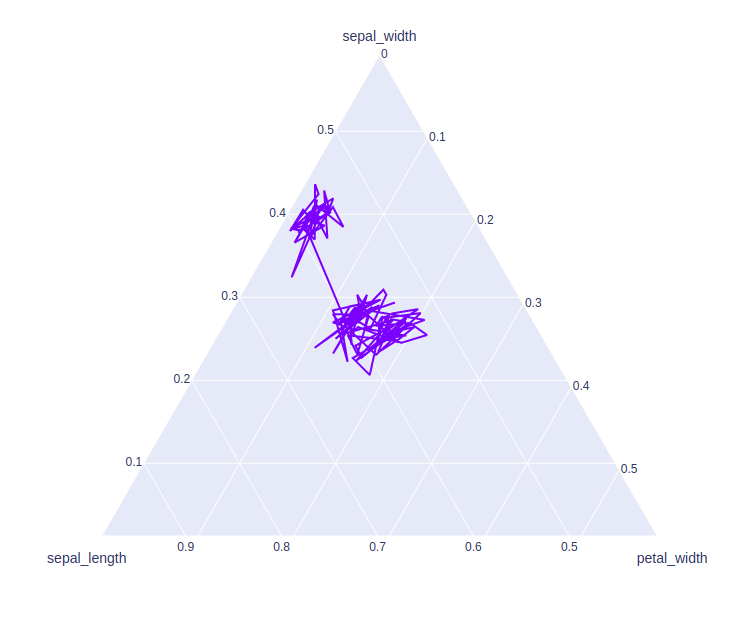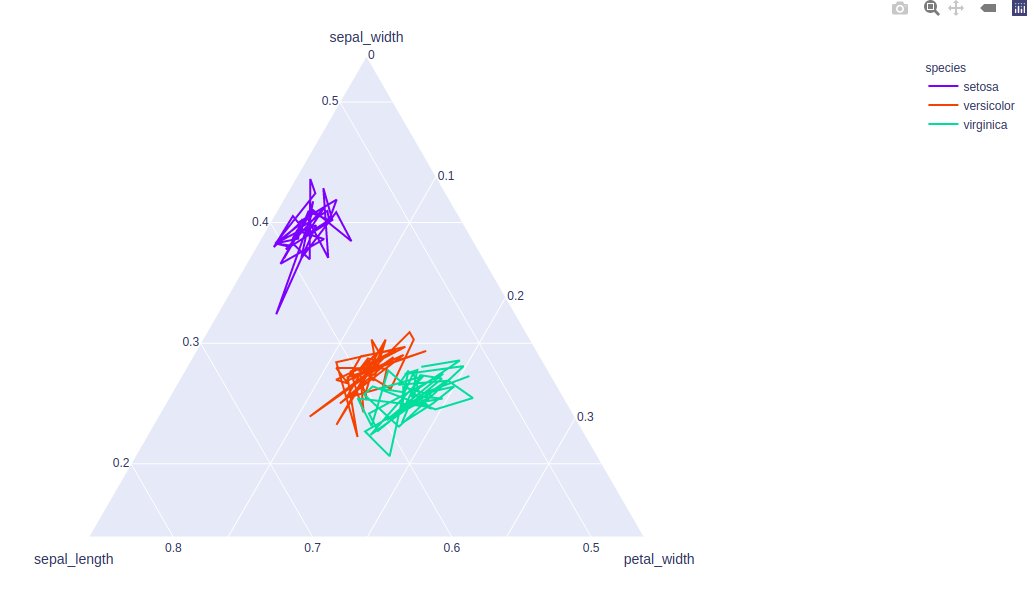plotly.express.line_ternary() function in Python

• Last Updated : 20 Jul, 2020

Plotly library of Python can be very useful for data visualization and understanding the data simply and easily. Plotly graph objects are a high-level interface to plotly which are easy to use.

plotly.express.line_ternary()

This method is used to create a ternary line plot. A ternary line plot is used to depicts the ratio of three variables on an equilateral triangle.

Syntax: plotly.express.line_ternary(data_frame=None, a=None, b=None, c=None, color=None, line_dash=None, line_group=None, hover_name=None, hover_data=None, title=None, template=None, width=None, height=None)

Parameters:

data_frame: DataFrame or array-like or dict needs to be passed for column names.

a, b, c:  These parameters are either a name of a column in data_frame, or a pandas Series or array_like object. These are used to position marks along the a, b, and c axis in ternary coordinates respectively.

color: This parameters assign color to marks.

hover_name:  Values from this column or array_like appear in bold in the hover tooltip.

hover_data: This parameter is used to appear in the hover tooltip or tuples with a bool or formatting string as first element, and list-like data to appear in hover as second element Values from these columns appear as extra data in the hover tooltip.

title: The title of the figure.

width: Set the width of the figure.

height: Set the height of the figure.

Example 1:

Python3

 import plotly.express as px  df = px.data.iris()  plot = px.line_ternary(df, a = 'sepal_width',                          b = 'sepal_length',                           c = 'petal_width')plot.show()

Output:Example 2: Using color and line_group parameter

Python3

 import plotly.express as px  df = px.data.iris()  plot = px.line_ternary(df, a = 'sepal_width',                          b = 'sepal_length',                           c = 'petal_width',                           color = 'species',                          line_group = 'species')plot.show()

Output:My Personal Notes arrow_drop_up